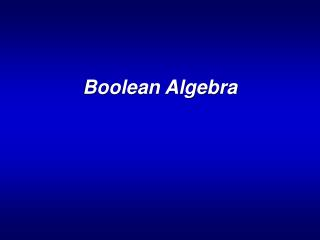DownloadDownload PresentationBoolean Algebra

# Boolean Algebra

Télécharger la présentation## Boolean Algebra

- - - - - - - - - - - - - - - - - - - - - - - - - - - E N D - - - - - - - - - - - - - - - - - - - - - - - - - - -
##### Presentation Transcript

1. Boolean Algebra

2. Boolean Algebra • A Boolean algebra • A set of operators (e.g. the binary operators: +, •, INV) • A set of axioms or postulates ENEE 644

3. Postulates • Commutative x+y = y+x x•y=y•x • Distributive x+(y•z)=(x+y)•(x+z) x•(y+z)=x•y+x•z • Identities x+0=x x•1=x • Complement x+x’=1 x•x’=0 Closure x+y x•y Associative (x+y)+z=x+(y+z) (x•y)•z=x•(y•z) ENEE 644

4. Properties of Boolean Algebra • Complement of a variable is unique. • (x’)’ = x -- involution • x+x = x --idempotent • x+1=1 • x+x•y=x -- absorption • (x+y)’=x’•y’ -- DeMorgan’s Law • xy+x’z+yz = xy + x’z -- consensus • Complement of a variable is unique. • (x’)’ = x -- involution • x+x = x x•x=x --idempotent • x+1=1 x•0=0 • x+x•y=x x•(x+y)=x -- absorption • (x+y)’=x’•y’ (x•y)’=x’+y’ -- DeMorgan’s Law • xy+x’z+yz = xy + x’z (x+y) •(x’+z) •(y+z) = (x+y) •(x’+z) -- consensus Duality: +  • and 0  1 ENEE 644

5. Proof of Consensus • a <= b essentially implies that if a =1 then b =1 and if a = 0 then b could be anything Theorem: In Consensus xy + x’z + yz = xy + x’z (How?) We Prove that xy + x’z + yz >= xy + x’z and xy + x’z + yz <= xy + x’z (This would imply equality and prove the theorem) Proof: First: xy + x’z + yz >= xy + x’z. Let xy + x’z = a and yz = b; So we want to prove that a + b >= a which is true by definition of the inequality Second: xy + x’z + yz <= xy + x’z. This inequality could be split into xy + x’z <= xy + x’z and yz <= xy + x’z All we need to do is to prove yz <= xy + x’z ENEE 644

6. Proof of Consensus yz <= xy + x’z We know that a <= b iff ab’ = 0 (How?) So if the above inequality is true yz(xy + x’z)’ = 0 must be true. Which is always true. Hence Proved. ENEE 644

7. Boolean Functions • A Boolean function is a mapping f(x): Bn B. • Constant function: f(x1,…,xn) = b. • Projection (to the i-th axis): f(x1,…,xn) = xi. • A Boolean function is complete if f(x) is defined for all xBn. Otherwise the point x that f(x) is not defined is called a don’t care condition. • Operations on Boolean functions: • Sum: (f+g)(x) = f(x) + g(x) • Product: (f•g)(x) = f(x)•g(x) • Complement: (f’)(x) = (f(x))’ ENEE 644

8. x z y z y x Representations of Boolean Functions • Algebraic expressions • f(x,y,z) = xy+z • Tabular forms • Venn diagrams • Cubical representations • Binary decision diagrams (BDD) • Algebraic expressions • f(x,y,z) = xy+z • Algebraic expressions • f(x,y,z) = xy+z • Tabular forms • Algebraic expressions • f(x,y,z) = xy+z • Tabular forms • Venn diagrams • Algebraic expressions • f(x,y,z) = xy+z • Tabular forms • Venn diagrams • Cubical representations ENEE 644

9. x z y z y x Representations of Boolean Functions • Algebraic expressions • f(x,y,z) = xy+z • Tabular forms • Venn diagrams • Cubical representations • Binary decision diagrams (BDD) ENEE 644

10. Boole’s Expansion Theorem • The cofactor of f(x1,…,xn) w.r.t. xi(or x’i) is a Boolean function , s.t. • Boole’s Expansion Theorem: ENEE 644

11. Boole’s Expansion: Examples • f(x) = x • f(1) + x’ • f(0) for single-variable x = (x + f(0)) • (x’+f(1)) (from duality) • f(x+y) • f(x’+y) = f(1) • f(y) • Define g(x,y) = f(x+y)f(x’+y) g(x,y) = xg(1,y) + x’g(0,y) = xf(1)f(y) + x’f(y)f(1) = f(1)f(y) • What if expand w.r.t variable y? f(x+y) • f(x’+y) =yf(1)f(1) + y’f(x)f(x’) = yf(1)+y’f(1)f(0) = f(1)(yf(1) + y’f(0)) =f(1)f(y) ENEE 644

12. x y x’ y Hardware? Delay? 1 y f f f f Boole’s Expansion: Examples ENEE 644

13. minterms discriminants Complete Expansion ENEE 644

14. Canonical Forms • A form is called canonical if the representation of the function in that form is unique. • Minterm Canonical Form • AND-OR circuits • Pro and Cons of Canonical Forms • Unique up to permutation • Inefficient ENEE 644

15. Normal (Standard) Forms • SOP (Disjunctive Normal Form) • A disjunction of product terms • A product term (1 is also considered a product term) • 0 • The Primary Objective During Logic Minimization is to Remove the Redundancy in the Representation. ENEE 644

16. x’yz xyz z xy’z y x xy’z’ Implicants • An implicant of a function is a product term that is included in the function. • An implicant is prime if it cannot be included in any other implicants. • A prime implicant is essential if it is the only one that includes a minterm. Example: f(x,y,z) = xy’ + yz xy(not I),xyz(I, not PI), xz(PI,not EPI), yz(EPI) ENEE 644

17. Specification for Incompleteness • A Boolean function is incomplete if f(x) is NOT defined for some xBn. Such point x is called a don’t care condition. • Tabular representation • {1-set, 0-set, don’t care set} • 1-set = {xy} • 0-set = {x’y’} • Don’t care set = {x’y, xy’} ENEE 644

18. {xy,x’y} {xy’,x’y’} Don’t Care Conditions • Satisfiability don’t cares of a subcircuit consist of all input patterns that will never occur. • Observability don’t cares of a subcircuit are the input patterns that represent situations when an output is not observed. Example: x y y=0 ENEE 644